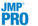Fitting Linear Models > Mixed Models > The Fit Mixed Report > Random Effects Covariance Parameter Estimates
Publication date: 07/30/2020

##Random Effects Covariance Parameter Estimates

The Random Effects Covariance Parameter Estimates report provides details for the covariance parameters of the random effects that you specified in the model.

Covariance Parameter

Lists all the covariance parameters of the random effects that you specified in the model.

Note: This column is labeled Variance Component when the model contains only variance components.

Subject

Lists the subject from which the block diagonal covariance matrix was formed.

Estimate

Gives the estimated variance or covariance component for the effect.

Note: When the model is equivalent to a REML model, a row for a Total covariance parameter is added to the table. The estimate for the Total covariance component is the sum of the positive variance components only.

Std Error

Gives the standard error for the covariance component estimate.

95% Lower

Gives the lower 95% confidence limit for the covariance component. See Confidence Intervals for Variance Components.

95% Upper

Gives the upper 95% confidence limit for the covariance component. See Confidence Intervals for Variance Components.

Wald p-Value

Gives the p-value for the test that the covariance parameter is equal to zero. This column appears only when you have selected Unbounded Variance Components in the Fit Model launch window.

###Confidence Intervals for Variance Components

The method used to calculate the confidence limits depends on whether you have selected Unbounded Variance Components in the Fit Model launch window. Note that the Unbounded Variance Components is selected by default.

If Unbounded Variance Components is selected, Wald-based confidence intervals are computed. These intervals are valid asymptotically, but note that they can be unreliable with small samples. The intervals are wider, which might lead you to mistakenly believe that an estimate is not significantly different from zero.

If Unbounded Variance Components is not selected, meaning that the parameters have a lower boundary constraint of zero, a Satterthwaite approximation is used (Satterthwaite 1946). The confidence intervals are also bounded at zero.